# 10 6 Volume of Prisms and Cylinders Warm

• Slides: 23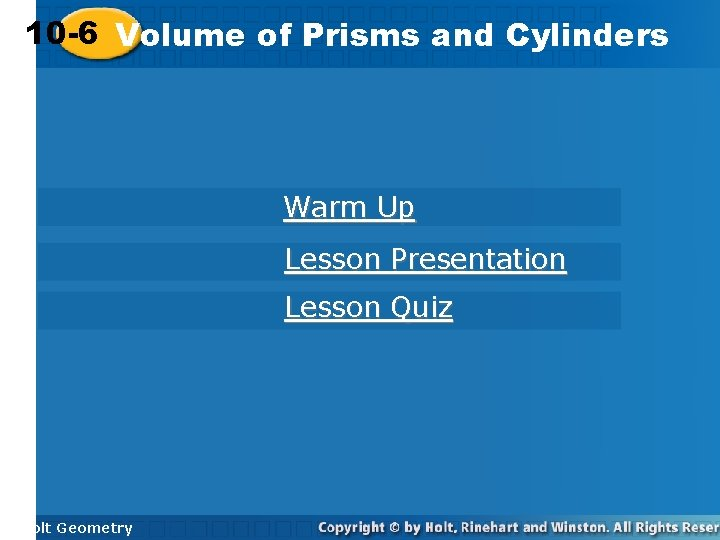10 -6 Volume of Prisms and Cylinders Warm Up Lesson Presentation Lesson Quiz Holt GeometryWarm Up Find the area of each figure. Round to the nearest tenth. 1. an equilateral triangle with edge length 20 cm 173. 2 cm 2 2. a regular hexagon with edge length 14 m 509. 2 m 2 3. a circle with radius 6. 8 in. 145. 3 in 2 4. a circle with diameter 14 ft 153. 9 ft 2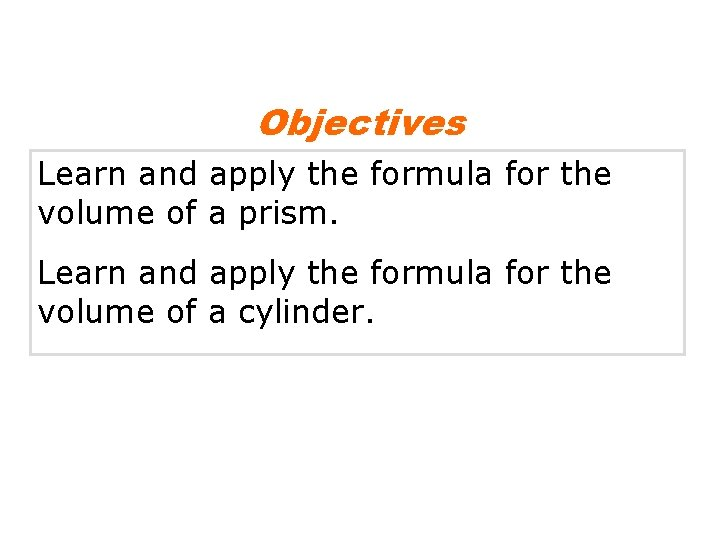Objectives Learn and apply the formula for the volume of a prism. Learn and apply the formula for the volume of a cylinder.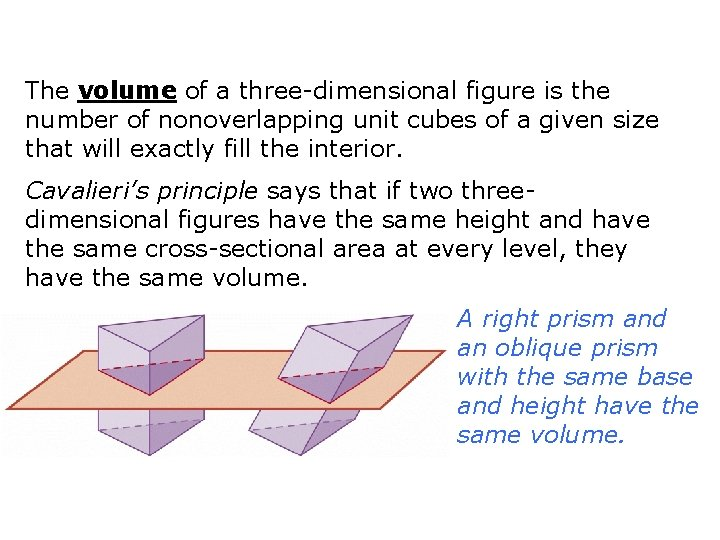The volume of a three-dimensional figure is the number of nonoverlapping unit cubes of a given size that will exactly fill the interior. Cavalieri’s principle says that if two threedimensional figures have the same height and have the same cross-sectional area at every level, they have the same volume. A right prism and an oblique prism with the same base and height have the same volume.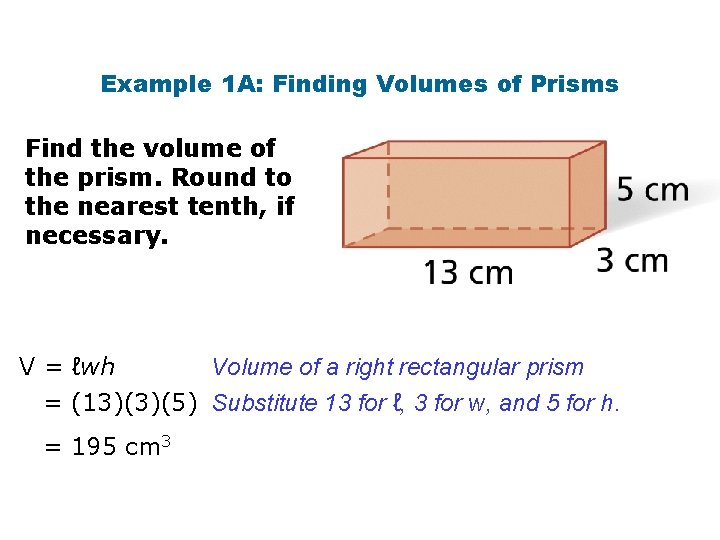Example 1 A: Finding Volumes of Prisms Find the volume of the prism. Round to the nearest tenth, if necessary. Volume of a right rectangular prism V = ℓwh = (13)(3)(5) Substitute 13 for ℓ, 3 for w, and 5 for h. = 195 cm 3Example 1 B: Finding Volumes of Prisms Find the volume of a cube with edge length 15 in. Round to the nearest tenth, if necessary. V = s 3 = (15)3 = 3375 in 3 Volume of a cube Substitute 15 for s.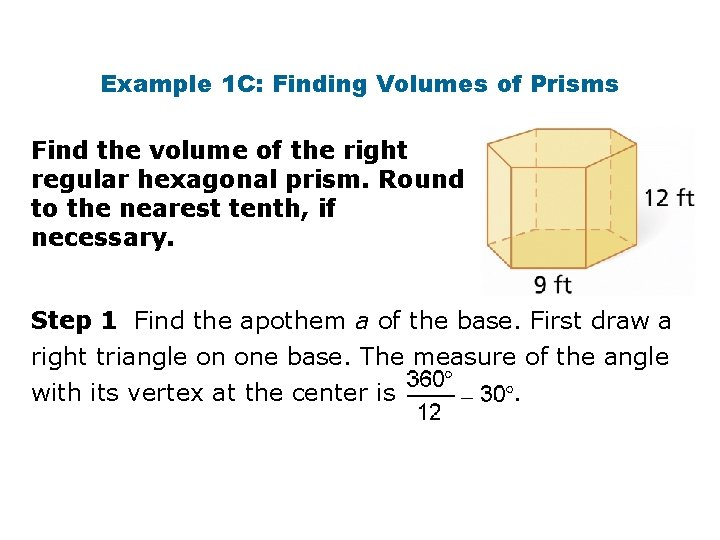Example 1 C: Finding Volumes of Prisms Find the volume of the right regular hexagonal prism. Round to the nearest tenth, if necessary. Step 1 Find the apothem a of the base. First draw a right triangle on one base. The measure of the angle with its vertex at the center is.Check It Out! Example 1 Find the volume of a triangular prism with a height of 9 yd whose base is a right triangle with legs 7 yd and 5 yd long. Volume of a triangular prism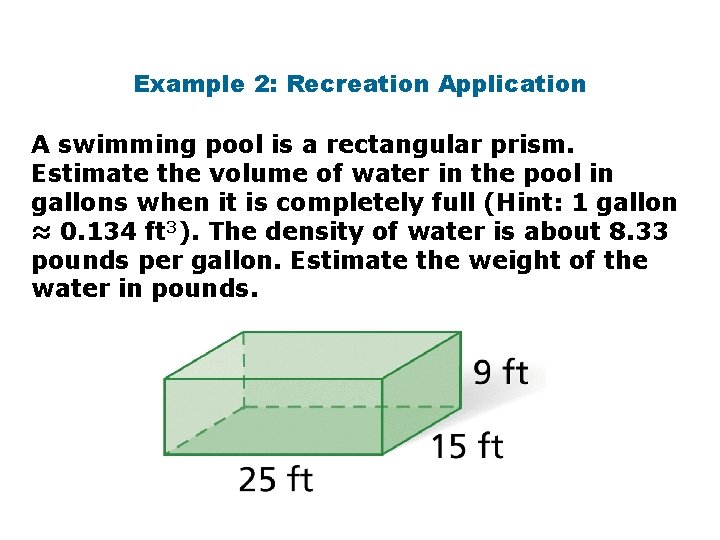Example 2: Recreation Application A swimming pool is a rectangular prism. Estimate the volume of water in the pool in gallons when it is completely full (Hint: 1 gallon ≈ 0. 134 ft 3). The density of water is about 8. 33 pounds per gallon. Estimate the weight of the water in pounds.Check It Out! Example 2 What if…? Estimate the volume in gallons and the weight of the water in the aquarium if the height were doubled. Step 1 Find the volume of the aquarium in cubic feet. V = ℓwh = (120)(60)(16) = 115, 200 ft 3Check It Out! Example 2 Continued What if…? Estimate the volume in gallons and the weight of the water in the aquarium if the height were doubled. The swimming pool holds about 859, 701 gallons. The water in the swimming pool weighs about 7, 161, 313 pounds.Cavalieri’s principle also relates to cylinders. The two stacks have the same number of CDs, so they have the same volume.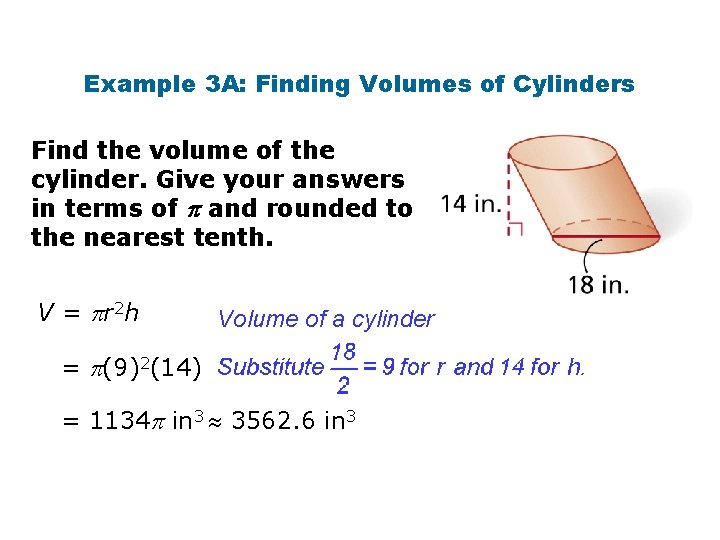Example 3 A: Finding Volumes of Cylinders Find the volume of the cylinder. Give your answers in terms of and rounded to the nearest tenth. V = r 2 h Volume of a cylinder = (9)2(14) = 1134 in 3 3562. 6 in 3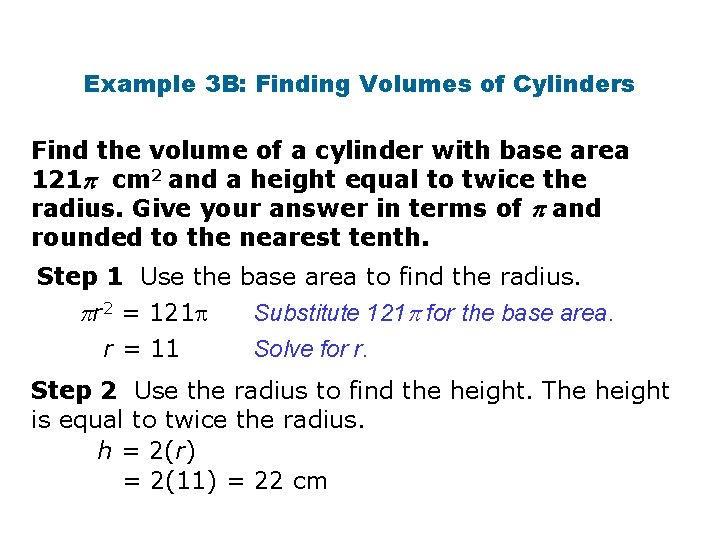Example 3 B: Finding Volumes of Cylinders Find the volume of a cylinder with base area 121 cm 2 and a height equal to twice the radius. Give your answer in terms of and rounded to the nearest tenth. Step 1 Use the base area to find the radius. Substitute 121 for the base area. r 2 = 121 Solve for r. r = 11 Step 2 Use the radius to find the height. The height is equal to twice the radius. h = 2(r) = 2(11) = 22 cmCheck It Out! Example 3 Find the volume of a cylinder with a diameter of 16 in. and a height of 17 in. Give your answer both in terms of π and rounded to the nearest tenth. V = r 2 h Volume of a cylinder = (8)2(17) Substitute 8 for r and 17 for h. = 1088 in 3 3418. 1 in 3Example 4: Exploring Effects of Changing Dimensions The radius and height of the cylinder are multiplied by. Describe the effect on the volume. original dimensions: radius and height multiplied by :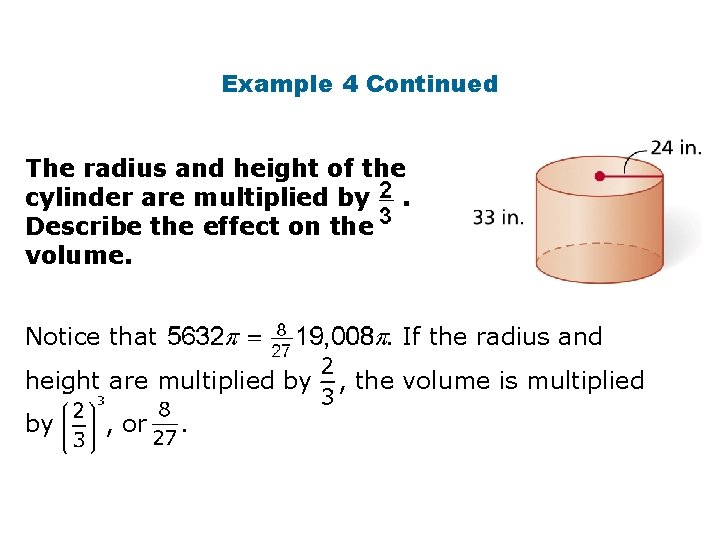Example 4 Continued The radius and height of the cylinder are multiplied by. Describe the effect on the volume. Notice that . If the radius and height are multiplied by by , or . , the volume is multiplied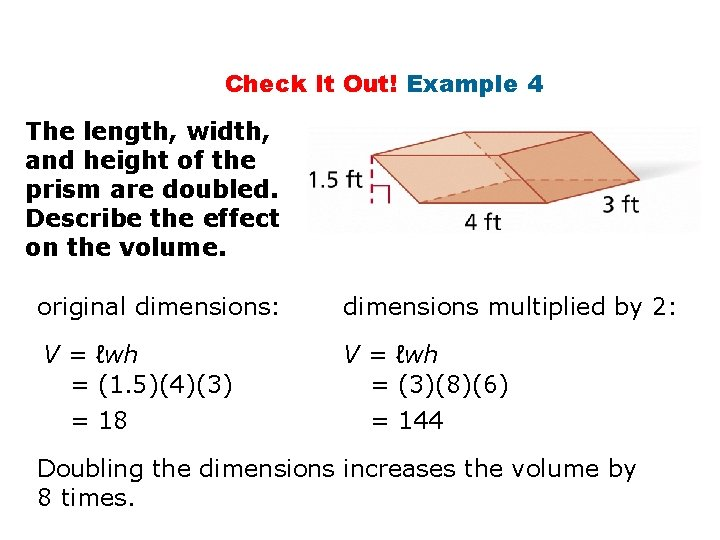Check It Out! Example 4 The length, width, and height of the prism are doubled. Describe the effect on the volume. original dimensions: dimensions multiplied by 2: V = ℓwh = (1. 5)(4)(3) = 18 V = ℓwh = (3)(8)(6) = 144 Doubling the dimensions increases the volume by 8 times.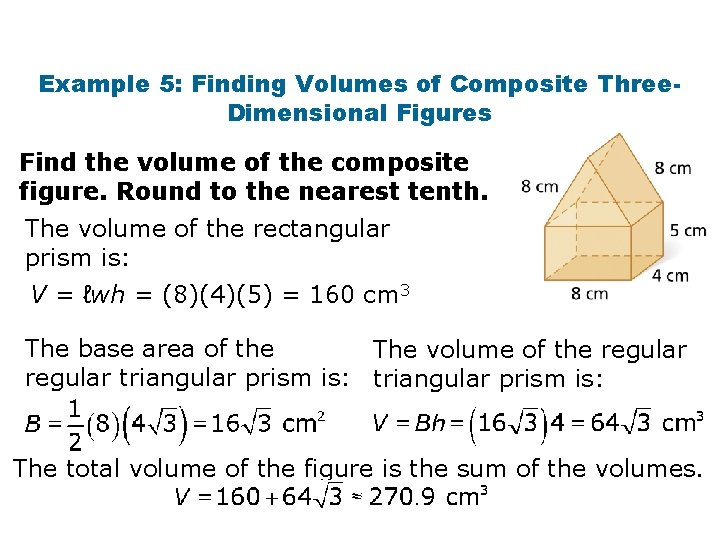Example 5: Finding Volumes of Composite Three. Dimensional Figures Find the volume of the composite figure. Round to the nearest tenth. The volume of the rectangular prism is: V = ℓwh = (8)(4)(5) = 160 cm 3 The base area of the The volume of the regular triangular prism is: The total volume of the figure is the sum of the volumes.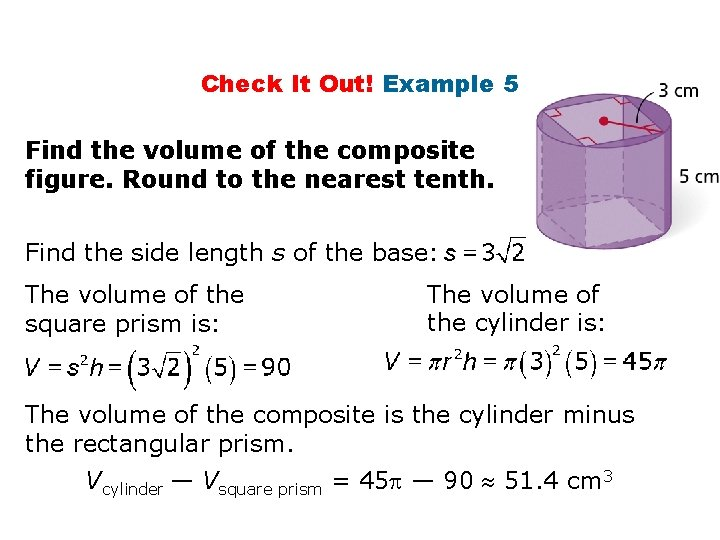Check It Out! Example 5 Find the volume of the composite figure. Round to the nearest tenth. Find the side length s of the base: The volume of the square prism is: The volume of the cylinder is: The volume of the composite is the cylinder minus the rectangular prism. Vcylinder — Vsquare prism = 45 — 90 51. 4 cm 3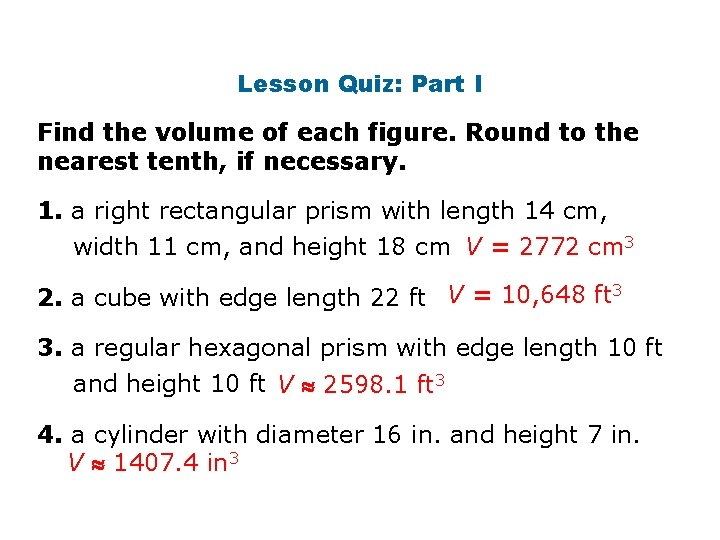Lesson Quiz: Part I Find the volume of each figure. Round to the nearest tenth, if necessary. 1. a right rectangular prism with length 14 cm, width 11 cm, and height 18 cm V = 2772 cm 3 2. a cube with edge length 22 ft V = 10, 648 ft 3 3. a regular hexagonal prism with edge length 10 ft and height 10 ft V 2598. 1 ft 3 4. a cylinder with diameter 16 in. and height 7 in. V 1407. 4 in 3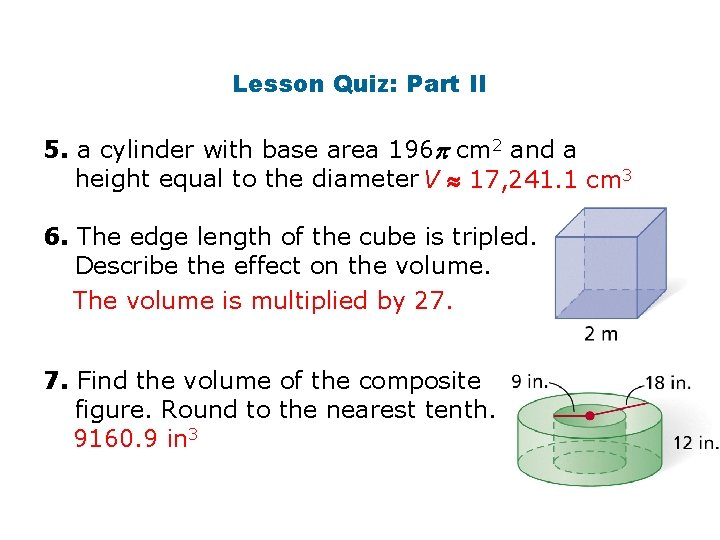Lesson Quiz: Part II 5. a cylinder with base area 196 cm 2 and a height equal to the diameter V 17, 241. 1 cm 3 6. The edge length of the cube is tripled. Describe the effect on the volume. The volume is multiplied by 27. 7. Find the volume of the composite figure. Round to the nearest tenth. 9160. 9 in 3